|

# CROX 闊合 |「多少」生活大師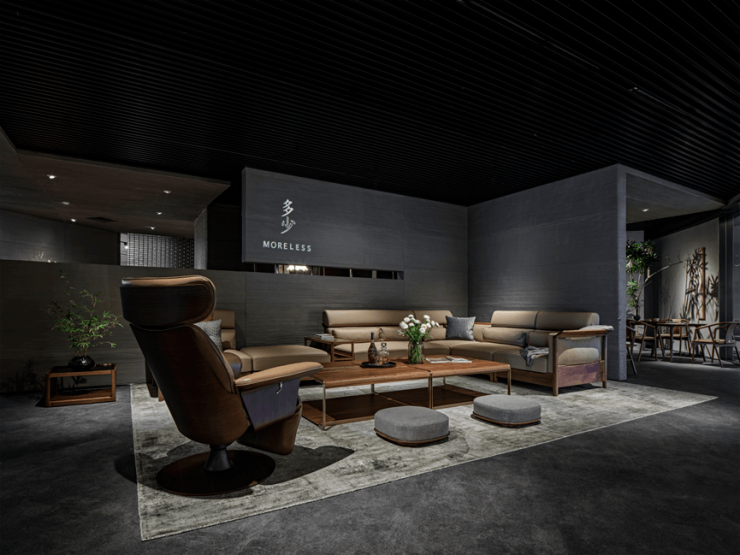△入口前厅

Located in the Foshan Life Master Collection, How Much Lecong Furniture Store continues How Much's brand philosophy of "Puddle is surplus, shabby is new, less is gain, more is confusing", hoping that this time, with a tiny and little space, we can cover the most diversified life styles by removing the complexity of simplicity, and using the "stacked and unpacked" as the inspiration to provide a space for people to gather, display, relax and communicate together. Crowe is inspired by the idea of "overlapping boxes" to provide a space for people to gather, display, relax and communicate with each other.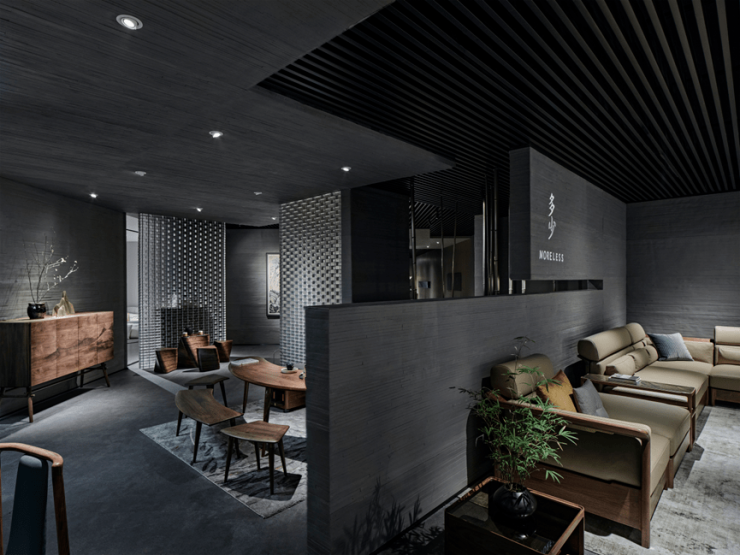△入口前厅一隅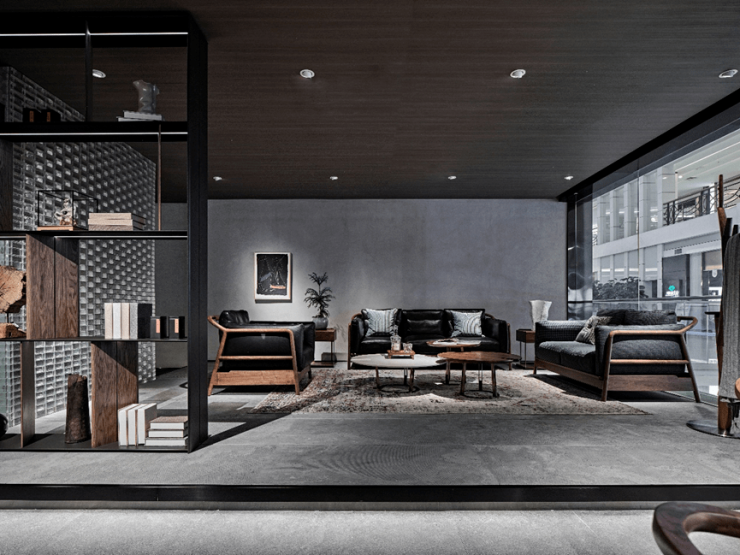△橱窗展示区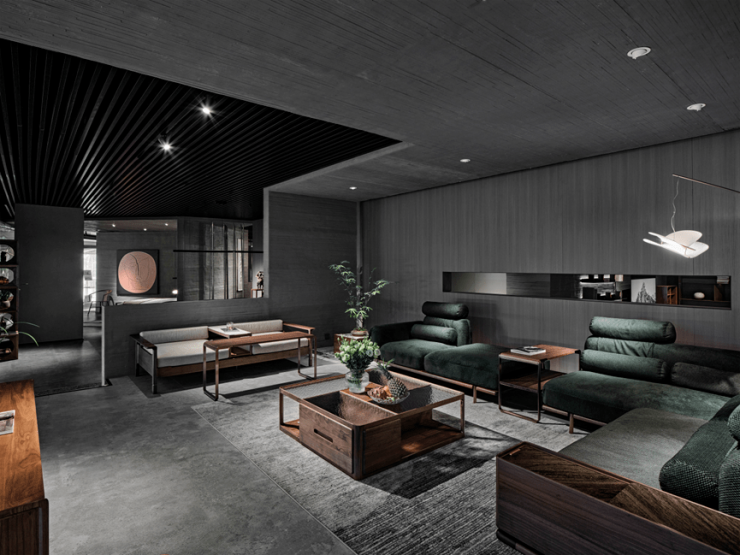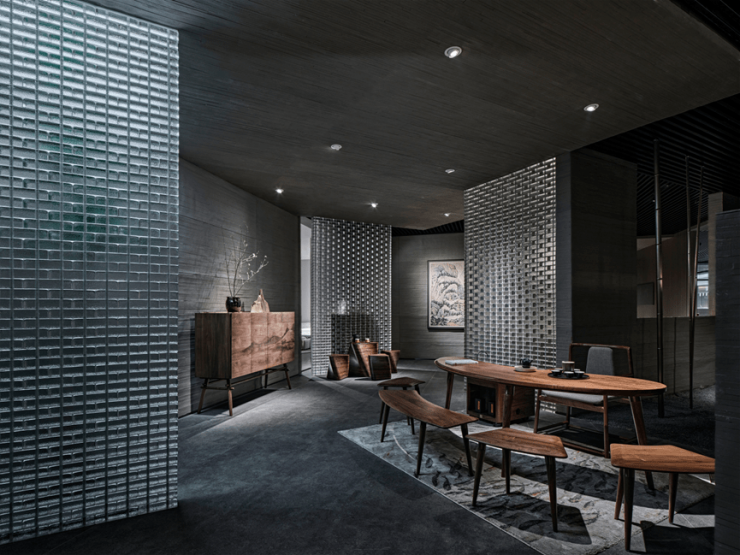△中庭转角处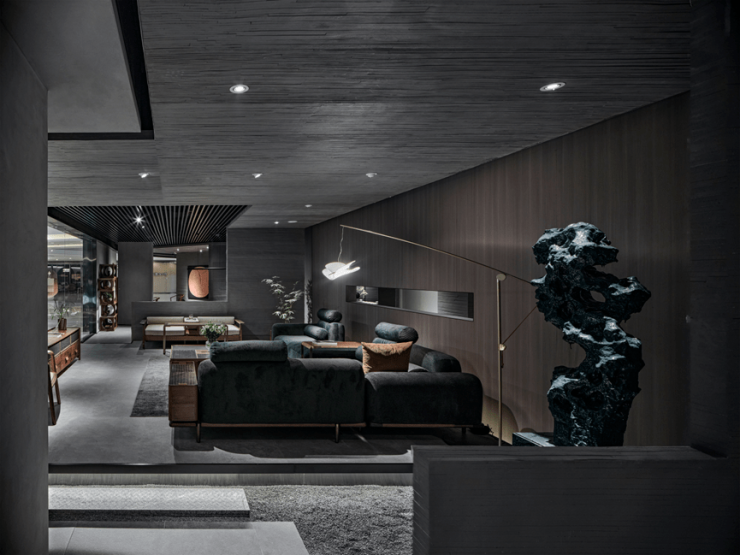△展陈洽谈空间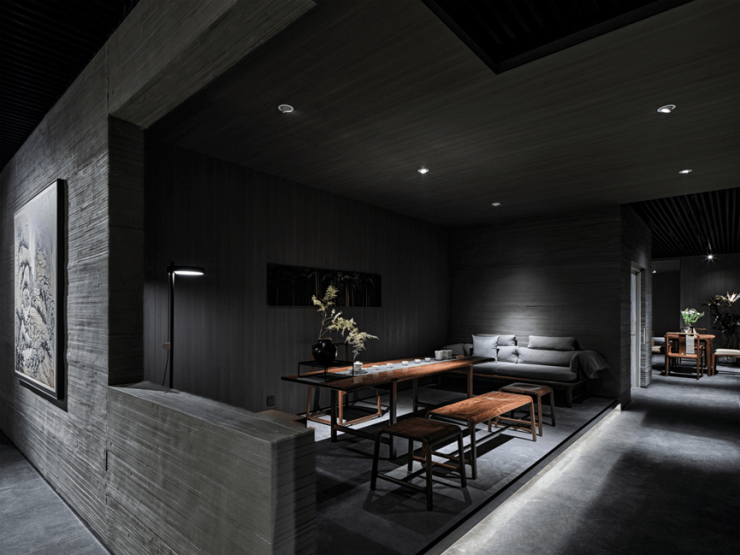△茶艺一角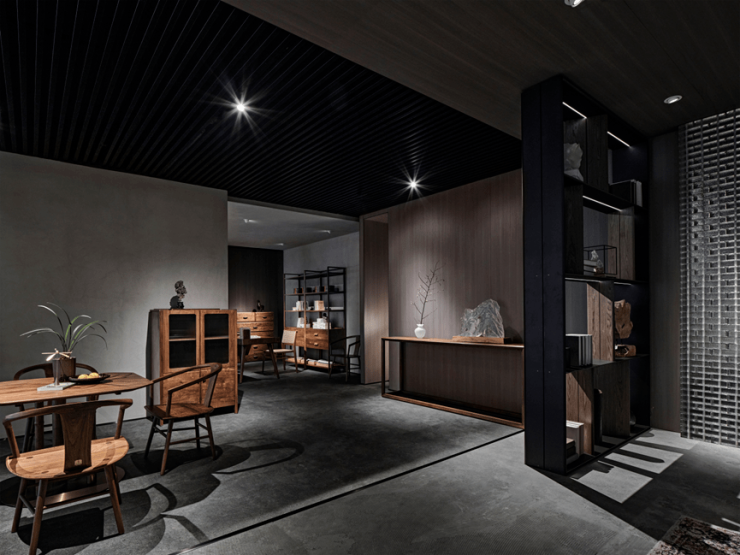△过度转换空间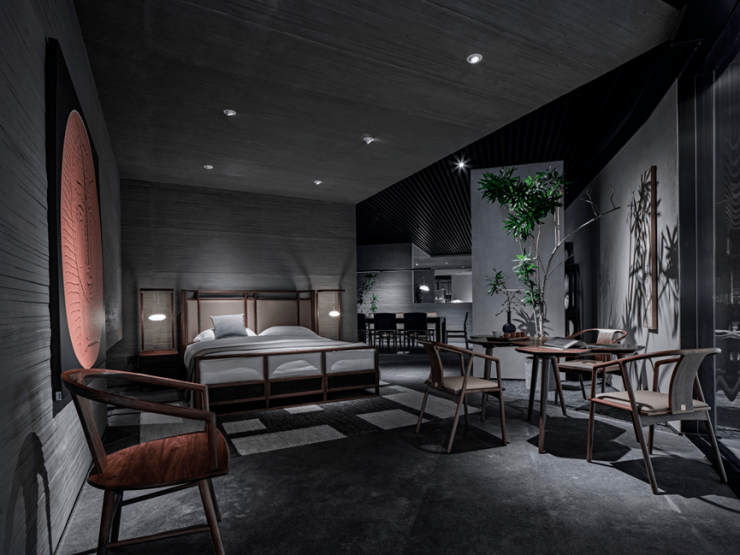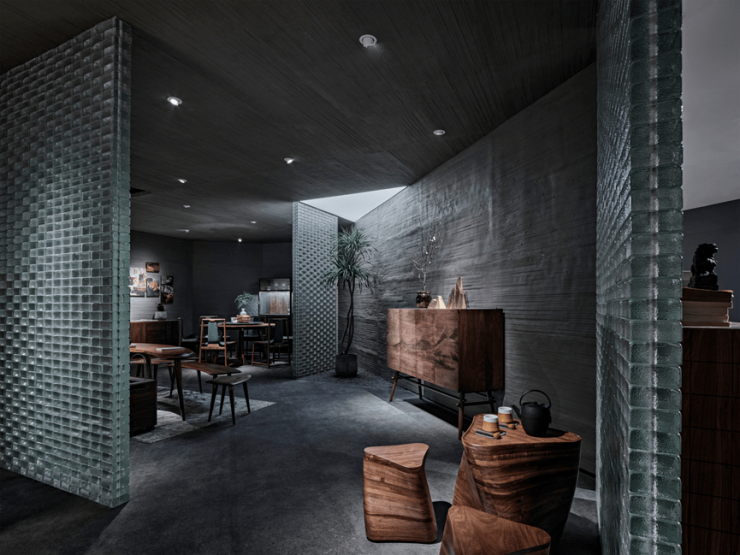At the beginning of the design, the box is opened with a happy and comfortable mind, and the spirit of the vessel is put into one piece of different "how many" artefacts, and the different needs of the scale of the trade-offs, to complete the portrayal of the future of the home. The final spatial layout is based on misplaced scenery, couplets and other techniques to create differences between individual areas, multi-level to express the life of less is more.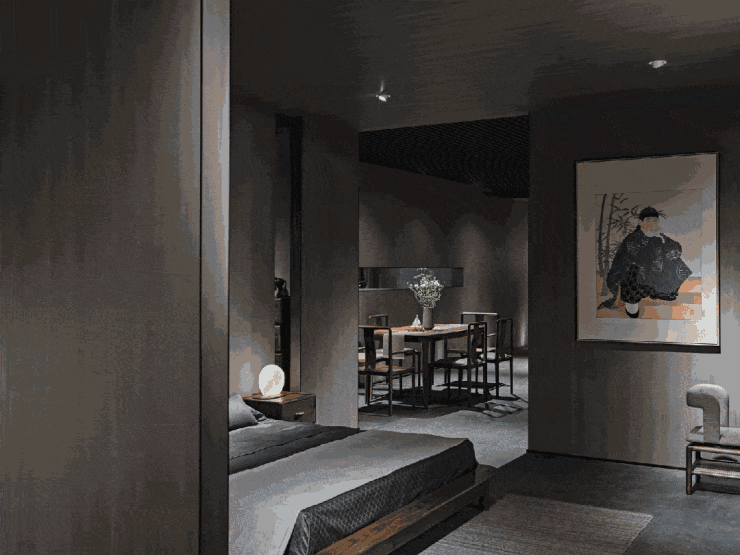The scale wanders back and forth between depth and breadth, where people go up and down, forward and backward, seemingly inside and outside, between the ability to bend and stretch, unfolding like a long scroll-like narrative of the tour, people follow the doorway of the hut, cut into the interior and then follow the winding paths formed by different spaces, freely travelling through the large and small independent scenes, the houses are independent of each other, and the space is unified and connected to each other, as if life is a combination of karma and interactions between each other to form a single body.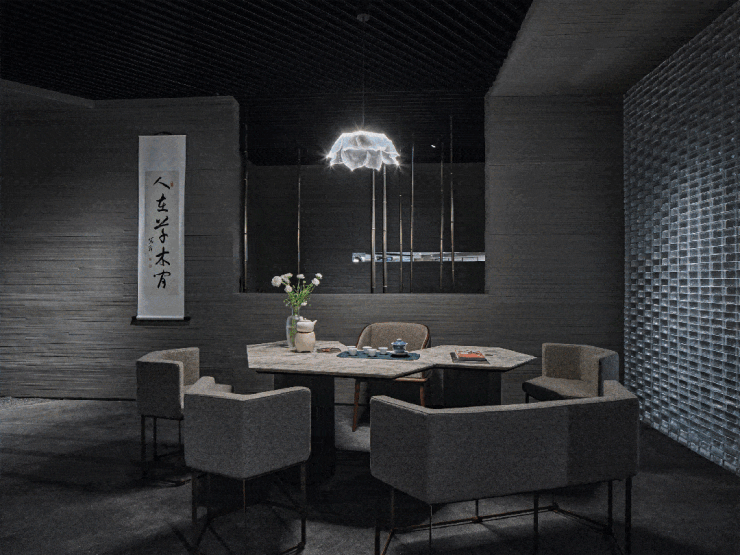From the exploration of contemporary Chinese life in the accidental, so that the mood of the space to form a kind of can open and close, changing the flow of the body, breaking free of the previous walls, roofs, the ground to intermittent cuts to break the integrity of the wooden cube, in the gaps implantation of plants, the introduction of light sources, to create different scales of scenery, but also the natural penetration of the natural existence of a loose or tight nature.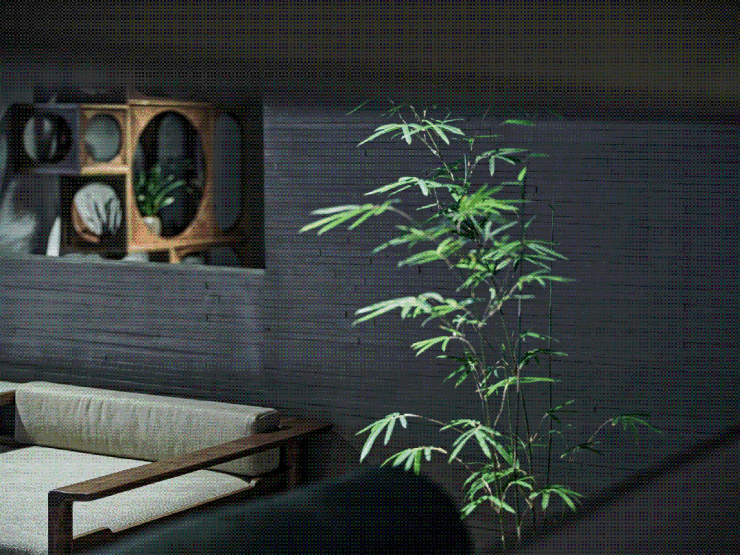Leisurely travelling to feel the freedom between the fragments here and there, is a continuation of the traditional life of writing, to do nothing for the freedom, is to create more or less of the beauty of contemporary naivety, life is a master, create nothing, to use.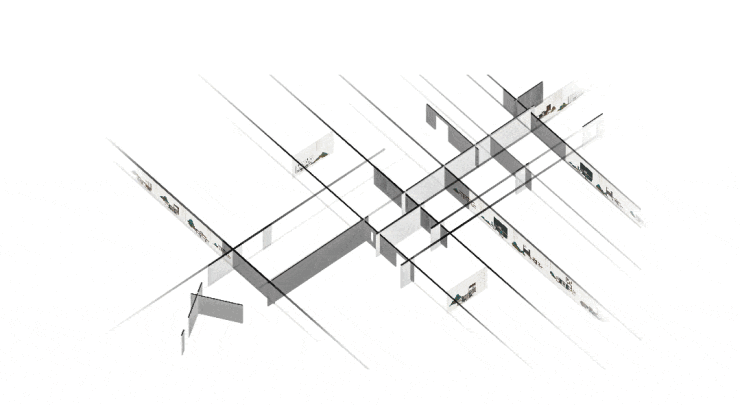△概念分析图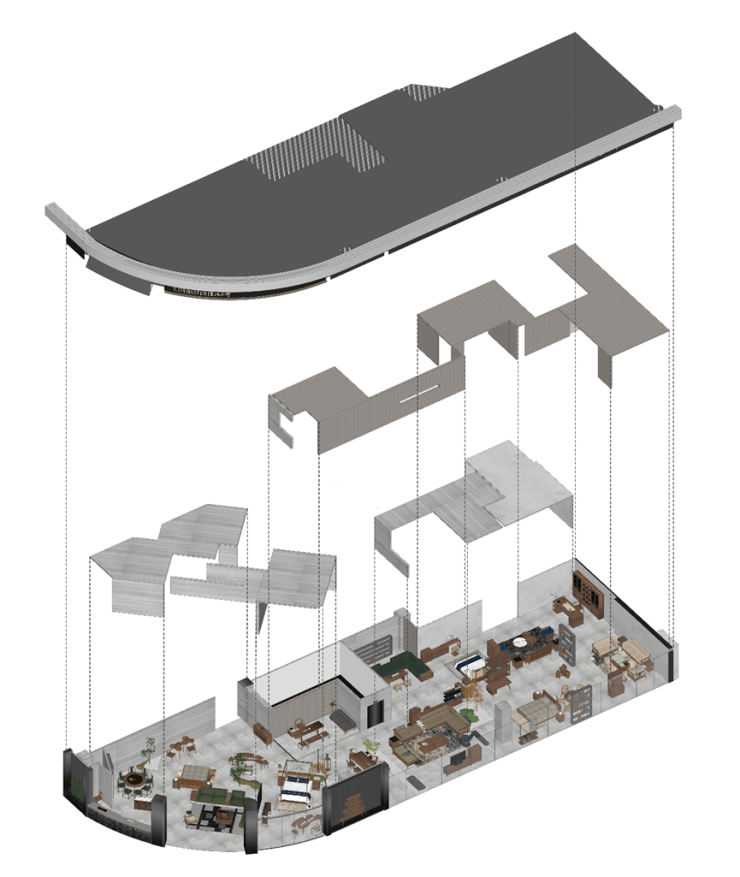Project Information

Project Name: "How Much" Life Master

Designed by: Wo Hop Crox

Design Team: Xu Chenxiang, Peng Rui, Zhu Yihan, Zheng Qingwen

Surface Area: 450 square metres

Completion date：March 2023

Building Material: Clear glass tiles, clear concrete, walnut wood, textured paint, black aluminium cube, etc.

Location: Foshan

Photography: Hu Wenjie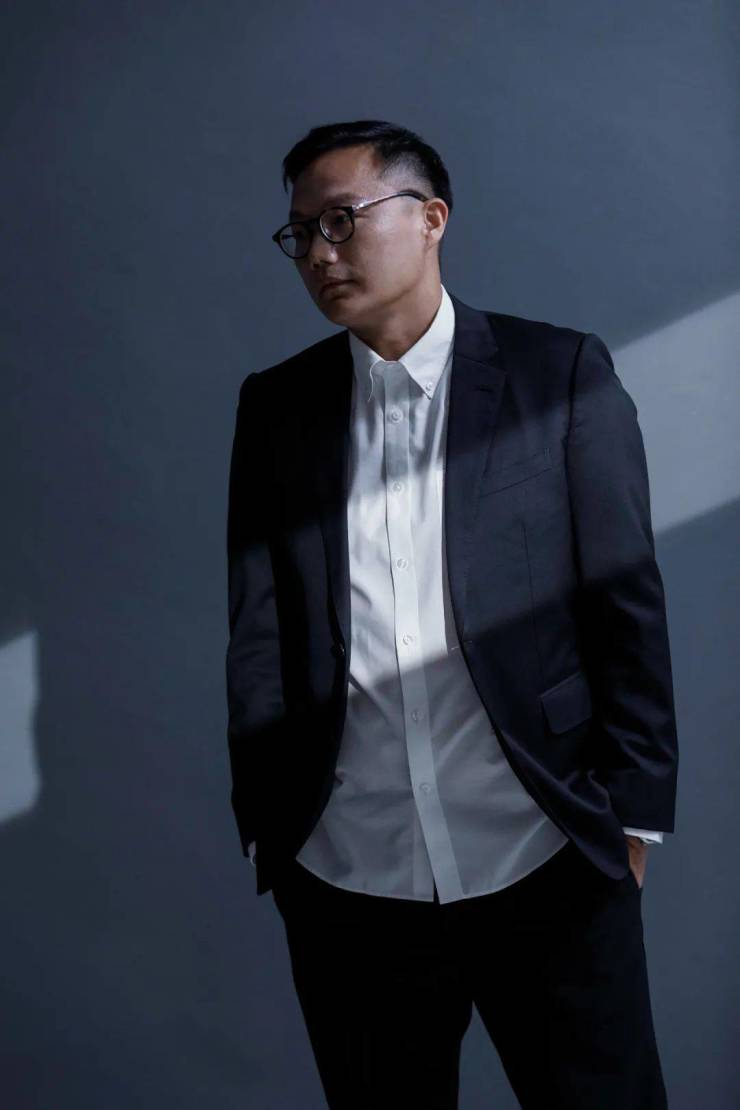CROX闊合创始人&设计总监

RIBA 英国皇家特许注册建筑师In CROX we believe design expands from the artistic feeling towards the conversation between humanism and nature. We bringing the intangible and organic imagination to achieve new life experiences.

`声明：本文由入驻焦点开放平台的作者撰写，除焦点官方账号外，观点仅代表作者本人，不代表焦点立场错误信息举报电话： 400-099-0099，邮箱：jubao@vip.sohu.com，或点此进行意见反馈，或点此进行举报投诉。`A B C D E F G H J K L M N P Q R S T W X Y Z
A - B - C - D - E
• A
• 鞍山
• 安庆
• 安阳
• 安顺
• 安康
• 澳门
• B
• 北京
• 保定
• 包头
• 巴彦淖尔
• 本溪
• 蚌埠
• 亳州
• 滨州
• 北海
• 百色
• 巴中
• 毕节
• 保山
• 宝鸡
• 白银
• 巴州
• C
• 承德
• 沧州
• 长治
• 赤峰
• 朝阳
• 长春
• 常州
• 滁州
• 池州
• 长沙
• 常德
• 郴州
• 潮州
• 崇左
• 重庆
• 成都
• 楚雄
• 昌都
• 慈溪
• 常熟
• D
• 大同
• 大连
• 丹东
• 大庆
• 东营
• 德州
• 东莞
• 德阳
• 达州
• 大理
• 德宏
• 定西
• 儋州
• 东平
• E
• 鄂尔多斯
• 鄂州
• 恩施
F - G - H - I - J
• F
• 抚顺
• 阜新
• 阜阳
• 福州
• 抚州
• 佛山
• 防城港
• G
• 赣州
• 广州
• 桂林
• 贵港
• 广元
• 广安
• 贵阳
• 固原
• H
• 邯郸
• 衡水
• 呼和浩特
• 呼伦贝尔
• 葫芦岛
• 哈尔滨
• 黑河
• 淮安
• 杭州
• 湖州
• 合肥
• 淮南
• 淮北
• 黄山
• 菏泽
• 鹤壁
• 黄石
• 黄冈
• 衡阳
• 怀化
• 惠州
• 河源
• 贺州
• 河池
• 海口
• 红河
• 汉中
• 海东
• 怀来
• I
• J
• 晋中
• 锦州
• 吉林
• 鸡西
• 佳木斯
• 嘉兴
• 金华
• 景德镇
• 九江
• 吉安
• 济南
• 济宁
• 焦作
• 荆门
• 荆州
• 江门
• 揭阳
• 金昌
• 酒泉
• 嘉峪关
K - L - M - N - P
• K
• 开封
• 昆明
• 昆山
• L
• 廊坊
• 临汾
• 辽阳
• 连云港
• 丽水
• 六安
• 龙岩
• 莱芜
• 临沂
• 聊城
• 洛阳
• 漯河
• 娄底
• 柳州
• 来宾
• 泸州
• 乐山
• 六盘水
• 丽江
• 临沧
• 拉萨
• 林芝
• 兰州
• 陇南
• M
• 牡丹江
• 马鞍山
• 茂名
• 梅州
• 绵阳
• 眉山
• N
• 南京
• 南通
• 宁波
• 南平
• 宁德
• 南昌
• 南阳
• 南宁
• 内江
• 南充
• P
• 盘锦
• 莆田
• 萍乡
• 平顶山
• 濮阳
• 攀枝花
• 普洱
• 平凉
Q - R - S - T - W
• Q
• 秦皇岛
• 齐齐哈尔
• 衢州
• 泉州
• 青岛
• 清远
• 钦州
• 黔南
• 曲靖
• 庆阳
• R
• 日照
• 日喀则
• S
• 石家庄
• 沈阳
• 双鸭山
• 绥化
• 上海
• 苏州
• 宿迁
• 绍兴
• 宿州
• 三明
• 上饶
• 三门峡
• 商丘
• 十堰
• 随州
• 邵阳
• 韶关
• 深圳
• 汕头
• 汕尾
• 三亚
• 三沙
• 遂宁
• 山南
• 商洛
• 石嘴山
• T
• 天津
• 唐山
• 太原
• 通辽
• 铁岭
• 泰州
• 台州
• 铜陵
• 泰安
• 铜仁
• 铜川
• 天水
• 天门
• W
• 乌海
• 乌兰察布
• 无锡
• 温州
• 芜湖
• 潍坊
• 威海
• 武汉
• 梧州
• 渭南
• 武威
• 吴忠
• 乌鲁木齐
X - Y - Z
• X
• 邢台
• 徐州
• 宣城
• 厦门
• 新余
• 新乡
• 许昌
• 信阳
• 襄阳
• 孝感
• 咸宁
• 湘潭
• 湘西
• 西双版纳
• 西安
• 咸阳
• 西宁
• 仙桃
• 西昌
• Y
• 阳泉
• 运城
• 营口
• 盐城
• 扬州
• 鹰潭
• 宜春
• 烟台
• 宜昌
• 岳阳
• 益阳
• 永州
• 阳江
• 云浮
• 玉林
• 宜宾
• 雅安
• 玉溪
• 延安
• 榆林
• 银川
• Z
• 张家口
• 镇江
• 舟山
• 漳州
• 淄博
• 枣庄
• 郑州
• 周口
• 驻马店
• 株洲
• 张家界
• 珠海
• 湛江
• 肇庆
• 中山
• 自贡
• 资阳
• 遵义
• 昭通
• 张掖
• 中卫

1室1厅1厨1卫1阳台

1
2
3
4
5

0
1
2

1

1

0
1
2
3报名成功，资料已提交审核A B C D E F G H J K L M N P Q R S T W X Y Z
A - B - C - D - E
• A
• 鞍山
• 安庆
• 安阳
• 安顺
• 安康
• 澳门
• B
• 北京
• 保定
• 包头
• 巴彦淖尔
• 本溪
• 蚌埠
• 亳州
• 滨州
• 北海
• 百色
• 巴中
• 毕节
• 保山
• 宝鸡
• 白银
• 巴州
• C
• 承德
• 沧州
• 长治
• 赤峰
• 朝阳
• 长春
• 常州
• 滁州
• 池州
• 长沙
• 常德
• 郴州
• 潮州
• 崇左
• 重庆
• 成都
• 楚雄
• 昌都
• 慈溪
• 常熟
• D
• 大同
• 大连
• 丹东
• 大庆
• 东营
• 德州
• 东莞
• 德阳
• 达州
• 大理
• 德宏
• 定西
• 儋州
• 东平
• E
• 鄂尔多斯
• 鄂州
• 恩施
F - G - H - I - J
• F
• 抚顺
• 阜新
• 阜阳
• 福州
• 抚州
• 佛山
• 防城港
• G
• 赣州
• 广州
• 桂林
• 贵港
• 广元
• 广安
• 贵阳
• 固原
• H
• 邯郸
• 衡水
• 呼和浩特
• 呼伦贝尔
• 葫芦岛
• 哈尔滨
• 黑河
• 淮安
• 杭州
• 湖州
• 合肥
• 淮南
• 淮北
• 黄山
• 菏泽
• 鹤壁
• 黄石
• 黄冈
• 衡阳
• 怀化
• 惠州
• 河源
• 贺州
• 河池
• 海口
• 红河
• 汉中
• 海东
• 怀来
• I
• J
• 晋中
• 锦州
• 吉林
• 鸡西
• 佳木斯
• 嘉兴
• 金华
• 景德镇
• 九江
• 吉安
• 济南
• 济宁
• 焦作
• 荆门
• 荆州
• 江门
• 揭阳
• 金昌
• 酒泉
• 嘉峪关
K - L - M - N - P
• K
• 开封
• 昆明
• 昆山
• L
• 廊坊
• 临汾
• 辽阳
• 连云港
• 丽水
• 六安
• 龙岩
• 莱芜
• 临沂
• 聊城
• 洛阳
• 漯河
• 娄底
• 柳州
• 来宾
• 泸州
• 乐山
• 六盘水
• 丽江
• 临沧
• 拉萨
• 林芝
• 兰州
• 陇南
• M
• 牡丹江
• 马鞍山
• 茂名
• 梅州
• 绵阳
• 眉山
• N
• 南京
• 南通
• 宁波
• 南平
• 宁德
• 南昌
• 南阳
• 南宁
• 内江
• 南充
• P
• 盘锦
• 莆田
• 萍乡
• 平顶山
• 濮阳
• 攀枝花
• 普洱
• 平凉
Q - R - S - T - W
• Q
• 秦皇岛
• 齐齐哈尔
• 衢州
• 泉州
• 青岛
• 清远
• 钦州
• 黔南
• 曲靖
• 庆阳
• R
• 日照
• 日喀则
• S
• 石家庄
• 沈阳
• 双鸭山
• 绥化
• 上海
• 苏州
• 宿迁
• 绍兴
• 宿州
• 三明
• 上饶
• 三门峡
• 商丘
• 十堰
• 随州
• 邵阳
• 韶关
• 深圳
• 汕头
• 汕尾
• 三亚
• 三沙
• 遂宁
• 山南
• 商洛
• 石嘴山
• T
• 天津
• 唐山
• 太原
• 通辽
• 铁岭
• 泰州
• 台州
• 铜陵
• 泰安
• 铜仁
• 铜川
• 天水
• 天门
• W
• 乌海
• 乌兰察布
• 无锡
• 温州
• 芜湖
• 潍坊
• 威海
• 武汉
• 梧州
• 渭南
• 武威
• 吴忠
• 乌鲁木齐
X - Y - Z
• X
• 邢台
• 徐州
• 宣城
• 厦门
• 新余
• 新乡
• 许昌
• 信阳
• 襄阳
• 孝感
• 咸宁
• 湘潭
• 湘西
• 西双版纳
• 西安
• 咸阳
• 西宁
• 仙桃
• 西昌
• Y
• 阳泉
• 运城
• 营口
• 盐城
• 扬州
• 鹰潭
• 宜春
• 烟台
• 宜昌
• 岳阳
• 益阳
• 永州
• 阳江
• 云浮
• 玉林
• 宜宾
• 雅安
• 玉溪
• 延安
• 榆林
• 银川
• Z
• 张家口
• 镇江
• 舟山
• 漳州
• 淄博
• 枣庄
• 郑州
• 周口
• 驻马店
• 株洲
• 张家界
• 珠海
• 湛江
• 肇庆
• 中山
• 自贡
• 资阳
• 遵义
• 昭通
• 张掖
• 中卫• 手机• 分享
• 设计
免费设计
• 计算器
装修计算器
• 联系
• 置顶
返回顶部